# NCERT Solutions class-11 Maths Miscellaneous## myCBSEguide App

CBSE, NCERT, JEE Main, NEET-UG, NDA, Exam Papers, Question Bank, NCERT Solutions, Exemplars, Revision Notes, Free Videos, MCQ Tests & more.

Miscellaneous Exercise

1. How many words, with or without meaning, each of 2 vowels and 3 consonants can be formed from the letters of the word DAUGHTER?

Ans. There are 8 letters in the word DAUGHTER. In this word 3 vowels and 5 consonants. 2 vowels and 3 consonants are to be selected.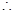Number of ways of selection =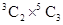=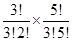=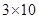= 30

Now, each word contains 5 letters which can be arranged among themselves 5! Ways.

Therefore, total number of words =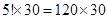= 3600

2. How many words, with or without meaning can be formed using all the letters of the word EQUATION at a time so that the vowels and consonants occur together?

Ans. There are 8 letters in the word EQUATION. In this word 5 vowels and 3 consonants. 2 vowels and 3 consonants are to be selected.

Now, 5 vowels can be arranged in 5! Ways and 3 consonants can be arranged in 3! Ways.

Also the groups of vowels and consonants can be arranged in 2! Ways.Total number of permutations =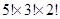=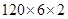= 1440

3. A committee of 7 has to be formed from 9 boys and 4 girls. In how many ways can this be done when the committee consists of:

(i) exactly 3 girls

(ii) at least 3 girls

(iii) almost 3 girls?

Ans. (i) There are 9 boys and 4 girls. 3 girls and 4 boys have to be selected.Number of ways of selection =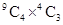=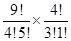=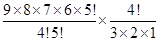= 504

(ii) We have to select at least 3 girls. So the committee consists of 3 girls and 4 boys or 4 girls and 3 boys.Number of ways of selection =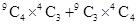=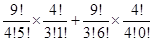=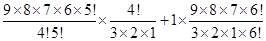= 504 + 84 = 588

(iii) We have to select at most 3 girls. So the committee consists of no girls and 7 boys or 1 girl and 6 boys or 2 girls and 5 boys or 3 girls and 4 boys.Number of ways of selection =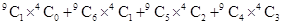=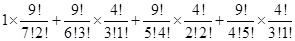=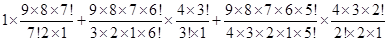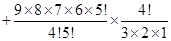= 36 + 336 + 756 + 504 = 1632

4. If the different permutations of all the letters of the word EXAMINATION are listed as in a dictionary, how many words are there in this list before the first word starting with E?

Ans. In the word EXAMINATION, there are two I’s and two N’s and all other letters are different.Number of ways of arrangement =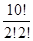=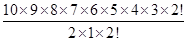= 907200

5. How many 6-digit numbers can be formed from the digits 0, 1 3, 5, 7 and 9 which are divisible by 10 and no digits are repeated?

Ans. A number divisible by 10 have unit place digit 0. So digit 0 is fixed at unit place and the remaining 5 placed filled with remaining five digits in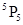ways.Required numbers =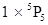=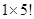= 120

6. The English alphabet has 5 vowels and 21 consonants. How many words with two different vowels and 2 different consonants can be formed from the alphabets?

Ans. 2 vowels out of 5 vowels and 2 consonants out of 21 consonants have to be selected and these 4 letters in 4 ways.Required number of words =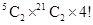=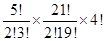=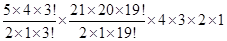=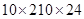= 50400

7. In an examination, a question paper consists of 12 questions divided into two parts i.e., part I and part II containing 5 and 7 questions, respectively. A student is required to attempt 8 questions in all, selecting at least 3 from each part. In how many ways can a student select the questions?

Ans. Here, we have to select 8 questions at least 3 questions from each section. Therefore, we have required selections are 3 from part I and 5 from part II or 4 from part I and 4 from part II or 5 from part I and 3 from part II.Number of ways of selection =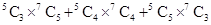=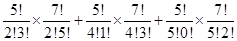=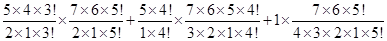=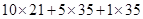= 210 + 175 + 35 = 420

8. Determine the number of 5-card combinations out of a deck of 52 cards is each selection of 5 cards has exactly one king.

Ans. Here, we have to select 5 cards containing 1 king and 4 other cards i.e., we have to select 1 king out of 4 kings and 4 other cards out of 48 other cards.Number of ways of selection =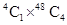=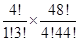=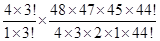=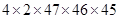= 778320

9. It is required to seat 5 men and 4 women in a row so that the women occupy the even places. How many such arrangements are possible?

Ans. Given: women occupy the even places.

1 2 3 4 5 6 7 8 9

M W M W M W M W M

Therefore, we can arrange four women in 4! Ways and 5 men in 5! Ways.Number of ways of selection =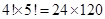= 2880

10. From a class of 25 students, 10 are to be chosen for an excursion party. There are 3 students who decide that either all of them will join or none of them will join. In how many ways can the excursion party be chosen?

Ans. According to the question, Number of ways of selection ==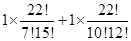=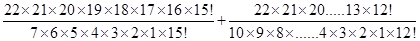= 170544 + 646646 = 817190

11. In how many ways can be letters of the word ASSASSINATION be arranged so that all the S’s are together?

Ans. In the word ASSASSINATION, A appears 3 times, S appears 4 times, I appears in 2 times and N appears in 2 times. Now, 4 S’ taken together become a single letter and other remaining letters taken with this single letter.

Number of arrangements =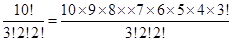= 151200### 1 thought on “NCERT Solutions class-11 Maths Miscellaneous”

1. Thank you so much for answer